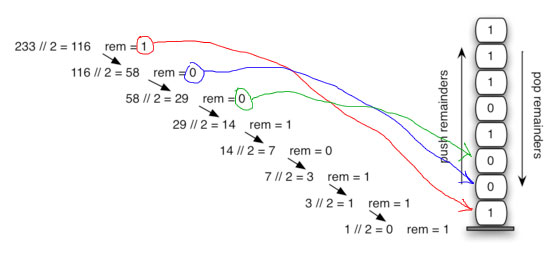TORONTO KIDS COMPUTER CLUB | PMCA Sunday 10:00 Java Homework 12.01
16797

# PMCA Sunday 10:00 Java Homework 12.01

## 04 Dec PMCA Sunday 10:00 Java Homework 12.01

Problem Description
We have learn how to use stack to solve some problems and how to convert decimal number into binary number:Please write the program to convert a given decimal number into a binary number by using the following steps for python coding solutions:

• takes an argument that is a decimal number and repeatedly divides it by 2.
• uses the built-in modulo operator, %, to extract the remainder and then pushes it on the stack.
• after the division process reaches 0, a binary string is constructed in the stack.
• creates an empty string.
• The binary digits are popped from the stack one at a time and appended to the right-hand end of the string.
• the binary string is then returned.

Sample Input:
241

Sample Output:
11110001